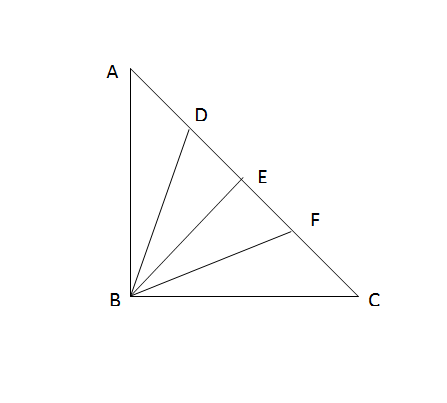# CAT Quantitative Aptitude Questions | Geometry Questions for CAT - Triangles

###### CAT Questions | CAT Geometry Questions | Triangles

The question is from the topic triangles. It tests the understanding of the basic concepts triangles. CAT Geometry questions are heavily tested in CAT exam. Make sure you master Geometry problems.

Question 43: In the below figure, ΔABC is right angled and AC = 100 cm. Also, AD = DE = EF = FC. Find the value of: BD2 + BE2 + BF2 (in cm2)1. 10,000 cm2
2. 5,000 cm2
3. 8,750 cm2
4. 12,500 cm2

## Best CAT Coaching in Chennai

#### CAT Coaching in Chennai - CAT 2022Limited Seats Available - Register Now!

##### Method of solving this CAT Question from Triangles: Apollonius’s theorem comes in handy in situations.E is the midpoint of hypotenuse AC so, BE = 1/2 ∗ AC=50 cm
Now, applying Apollonius’s theorem on ΔABE
=> AB2 + BE2 = 2 * (BD2 + AD2)
=> AB2 + 502 = 2 * (BD2 + 252)……….(1) (As, AD = DE = EF = FC = 25 cm)

Similarly, in ΔBEC,
=> BC2 + BE2 = 2 * (BF2 + EF2)
=> BC2 + 502 = 2 * (BF2 + 252)………..(2)

=> AB2 + BC2 + 2 * 502 = 2 * (BD2 + BF2 + 2 * 252)
=> AC2 + 2 * 502 = 2 * (BD2 + BF2) + 4 * 252 (As, AB2 + BC2 = AC2)
=> 1002 + 2 * 502 – 502 = 2 * (BD2 + BF2)
=> 1002+ 502 = 2 * (BD2 + BF2)
=> (10,000 + 2500)/2 = (BD2 + BF2)
=> 12500/2 = BD2 + BF2
Therefore, BD2 + BF2 = 6250
=> BD2 + BF2 + BE2 = 6250 + 502 = 6250 + 2500 = 8750 cm2

The question is "Find the value of: BD2 + BE2 + BF2 (in cm2)"

##### Hence, the answer is 8,750 cm2.

Choice C is the correct answer.

###### CAT Coaching in ChennaiCAT 2023

Classroom Batches Starting Now! @Gopalapuram

###### Best CAT Coaching in Chennai Introductory offer of 5000/-

Attend a Demo Class

## CAT Preparation Online | CAT Geometry questions Videos On YouTube

#### Other useful sources for Geometry Question | Geometry Triangles Circles Quadrilaterals Sample Questions

##### Where is 2IIM located?

2IIM Online CAT Coaching
A Fermat Education Initiative,
58/16, Indira Gandhi Street,
Kaveri Rangan Nagar, Saligramam, Chennai 600 093

##### How to reach 2IIM?

Phone: (91) 44 4505 8484
Mobile: (91) 99626 48484
WhatsApp: WhatsApp Now
Email: prep@2iim.com